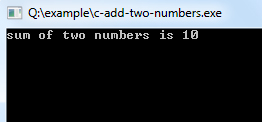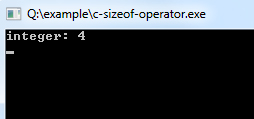# C Operators

C operators are symbols that are used to perform mathematical or logical manipulations. The C programming language is rich with built-in operators. Operators take part in a program for manipulating data and variables and form a part of the mathematical or logical expressions.

## Types of Operators in C

C programming language offers various types of operators having different functioning capabilities.
1. Arithmetic Operators
2. Relational Operators
3. Logical Operators
4. Assignment Operators
5. Increment and Decrement Operators
6. Conditional Operator
7. Bitwise Operators
8. Special Operators

## Arithmetic Operators

Arithmetic Operators are used to performing mathematical calculations like addition (+), subtraction (-), multiplication (*), division (/) and modulus (%).

OperatorDescription
-Subtraction
*Multiplication
/Division
%Modulus

### C Program to Add Two Numbers

Example:
``````#include <stdio.h>
void main()
{
int i=3,j=7,k; /* Variables Defining and Assign values */
k=i+j;
printf("sum of two numbers is %d\n", k);
}
``````
Program Output:## Increment and Decrement Operators

Increment and Decrement Operators are useful operators generally used to minimize the calculation, i.e. ++x and x++ means x=x+1 or -x and x−−means x=x-1. But there is a slight difference between ++ or −− written before or after the operand. Applying the pre-increment first add one to the operand and then the result is assigned to the variable on the left whereas post-increment first assigns the value to the variable on the left and then increment the operand.

OperatorDescription
++Increment
−−Decrement
Example: To Demonstrate prefix and postfix modes.
``````#include <stdio.h>
//stdio.h is a header file used for input.output purpose.

void main()
{
//set a and b both equal to 5.
int a=5, b=5;

//Print them and decrementing each time.
//Use postfix mode for a and prefix mode for b.
printf("\n%d %d",a--,--b);
printf("\n%d %d",a--,--b);
printf("\n%d %d",a--,--b);
printf("\n%d %d",a--,--b);
printf("\n%d %d",a--,--b);
}``````
Program Output:
```5 4
4 3
3 2
2 1
1 0```

## Relational Operators

Relational operators are used to comparing two quantities or values.

OperatorDescription
==Is equal to
!=Is not equal to
>Greater than
<Less than
>=Greater than or equal to
<=Less than or equal to

## Logical Operators

C provides three logical operators when we test more than one condition to make decisions. These are: && (meaning logical AND), || (meaning logical OR) and ! (meaning logical NOT).

OperatorDescription
&&And operator. It performs logical conjunction of two expressions. (if both expressions evaluate to True, result is True. If either expression evaluates to False, the result is False)
||Or operator. It performs a logical disjunction on two expressions. (if either or both expressions evaluate to True, the result is True)
!Not operator. It performs logical negation on an expression.

## Bitwise Operators

C provides a special operator for bit operation between two variables.

OperatorDescription
<<Binary Left Shift Operator
>>Binary Right Shift Operator
~Binary Ones Complement Operator
&Binary AND Operator
^Binary XOR Operator
|Binary OR Operator

## Assignment Operators

Assignment operators applied to assign the result of an expression to a variable. C has a collection of shorthand assignment operators.

OperatorDescription
=Assign
+=Increments then assign
-=Decrements then assign
*=Multiplies then assign
/=Divides then assign
%=Modulus then assign
<<=Left shift and assign
>>=Right shift and assign
&=Bitwise AND assign
^=Bitwise exclusive OR and assign
|=Bitwise inclusive OR and assign

## Conditional Operator

C offers a ternary operator which is the conditional operator (?: in combination) to construct conditional expressions.

OperatorDescription
? : Conditional Expression

## Special Operators

C supports some special operators

OperatorDescription
sizeof() Returns the size of a memory location.
& Returns the address of a memory location.
* Pointer to a variable.

### Program to demonstrate the use of sizeof operator

Example:
``````#include <stdio.h>
void main()
{
int i=10; /* Variables Defining and Assign values */      printf("integer: %d\n", sizeof(i));
}
``````
Program Output: